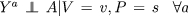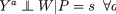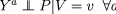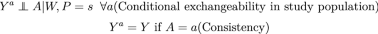Springer Nature is making SARS-CoV-2 and COVID-19 research free. View research | View latest news | Sign up for updates

# Effect heterogeneity and variable selection for standardizing causal effects to a target population

## Abstract

The participants in randomized trials and other studies used for causal inference are often not representative of the populations seen by clinical decision-makers. To account for differences between populations, researchers may consider standardizing results to a target population. We discuss several different types of homogeneity conditions that are relevant for standardization: Homogeneity of effect measures, homogeneity of counterfactual outcome state transition parameters, and homogeneity of counterfactual distributions. Each of these conditions can be used to show that a particular standardization procedure will result in an unbiased estimate of the effect in the target population, given assumptions about the relevant scientific context. We compare and contrast the homogeneity conditions, in particular their implications for selection of covariates for standardization and their implications for how to compute the standardized causal effect in the target population. While some of the recently developed counterfactual approaches to generalizability rely upon homogeneity conditions that avoid many of the problems associated with traditional approaches, they often require adjustment for a large (and possibly unfeasible) set of covariates.

This is a preview of subscription content, log in to check access.

## References

1. 1.

Weiss NS. Generalizing from the results of randomized studies of treatment: can non-randomized studies be of help? Eur J Epidemiol. 2019;34(8):715–8.

2. 2.

Hernán MA, Hernández-Díaz S, Robins JM. A structural approach to selection bias. Epidemiology (Cambridge, Mass.). 2004;15(5):615–25.

3. 3.

Suzuki E, Tsuda T, Mitsuhashi T, Mansournia MA, Yamamoto E. Errors in causal inference: an organizational schema for systematic error and random error. Ann Epidemiol. 2016;26(11):788–93.

4. 4.

Huitfeldt A, Goldstein A, Swanson SA. The choice of effect measure for binary outcomes: introducing counterfactual outcome state transition parameters. Epidemiol Methods. 2018;7(1):14.

5. 5.

Dahabreh IJ, Hernán MA. Extending inferences from a randomized trial to a target population. Eur J Epidemiol. 2019;34(8):719–22.

6. 6.

VanderWeele TJ. Confounding and effect modification: distribution and measure. Epidemiol Methods. 2012;1(1):55–82.

7. 7.

Greenland S. Interpretation and estimation of summary ratios under heterogeneity. Stat Med. 1982;1(3):217–27.

8. 8.

Bernard S, Neville KA, Nguyen AT, Flockhart DA. Interethnic differences in genetic polymorphisms of CYP2d6 in the U.S. population: clinical implications. Oncologist. 2006;11(2):126–35.

9. 9.

Huitfeldt A, Stensrud MJ, Suzuki E. On the collapsibility of measures of effect in the counterfactual causal framework. Emerg Themes Epidemiol. 2019;16(1):1–5.

10. 10.

Cochran WG. The comparison of percentages in matched samples. Biometrika. 1950;37(3/4):256–66.

11. 11.

Higgins JPT, Thompson SG, Deeks JJ, Altman DG. Measuring inconsistency in meta-analyses. BMJ Br Med J. 2003;327(7414):557–60.

12. 12.

Iwasaki M, Yamamoto S, Otani T, Inoue M, Hanaoka T, Sobue T, Tsugane S, Japan Public Health Center-based Prospective Study (JPHC Study) Group. Generalizability of relative risk estimates from a well-defined population to a general population. Eur J Epidemiol. 2006;21(4):253–62.

13. 13.

Poole C, Shrier I, VanderWeele TJ. Is the risk difference really a more heterogeneous measure? Epidemiology. 2015;26(5):714–8.

14. 14.

Spiegelman D, VanderWeele TJ. Evaluating public health interventions: 6. modeling ratios or differences? Let the data tell us. Am J Public Health. 2017;107(7):1087–91.

15. 15.

The European Network of Centres for Pharmacoepidemiology and Pharmacovigilance (ENCePP). Guide on Methodological Standards in Pharmacoepidemiology (Revision 7). EMA/95098/2010.

16. 16.

Sheps MC. Shall we count the living of the dead? N Engl J Med. 1958;259(25):1210–4.

17. 17.

Deeks JJ. Issues in the selection of a summary statistic for meta-analysis of clinical trials with binary outcomes. Stat Med. 2002;21(11):1575–600.

18. 18.

Baker R, Jackson D. A new measure of treatment effect for random-effects meta-analysis of comparative binary outcome data. 2018. ArXiv:1806.03471.

19. 19.

Deeks JJ, Higgins JPT, Altman DG (editors). Chapter 9: analysing data and undertaking meta-analyses. In: Higgins JPT, Green S, editors. Handbook for systematic reviews of interventions version 5.1.0 (updated March 2011). New York: Wiley; 2011.

20. 20.

Glasziou PP, Irwig LM. An evidence based approach to individualising treatment. BMJ. 1995;311(7016):1356–9.

21. 21.

Lesko CR, Buchanan AL, Westreich D, Edwards JK, Hudgens MG, Cole SR. Generalizing study results: a potential outcomes perspective. Epidemiology. 2017;28:553–61.

22. 22.

Murray EJ, Robins JM, Seage GR, Freedberg KA, Hernán MA. A comparison of agent-based models and the parametric G-formula for causal inference. Am J Epidemiol. 2017;186(2):131–42.

23. 23.

Stuart EA, Cole SR, Bradshaw CP, Leaf PJ. The use of propensity scores to assess the generalizability of results from randomized trials. J R Stat Soc Ser A Stat Soc. 2001;174(2):369–86.

24. 24.

Cole SR, Stuart EA. Generalizing evidence from randomized clinical trials to target populations: the ACTG 320 trial. Am J Epidemiol. 2010;172(1):107–15.

25. 25.

Horvitz DG, Thompson DJ. A generalization of sampling without replacement from a finite universe. J Am Stat Assoc. 1952;47(260):663–85.

26. 26.

Robins JM. Association, causation, and marginal structural models. Synthese. 1999;121(1–2):151–79.

27. 27.

Robins JM, Hernán MA, Brumback B. Marginal structural models and causal inference in epidemiology. Epidemiology (Cambridge, Mass.). 2000;11(5):550–60.

28. 28.

Westreich D, Edwards JK, Lesko CR, Stuart E, Cole SR. Transportability of trial results using inverse odds of sampling weights. Am J Epidemiol. 2017;186(8):1010–4.

29. 29.

Lu H, Cole SR, Hall HI, Schisterman EF, Breger TL, Edwards JK, Westreich D. Generalizing the per-protocol treatment effect: the case of ACTG A5095. Clin Trials. 2018;16(1):52–62.

30. 30.

Susukida R, Crum RM, Ebnesajjad C, Stuart EA, Mojtabai R. Generalizability of findings from randomized controlled trials: application to the National Institute of Drug Abuse Clinical Trials Network. Addiction. 2017;112(7):1210–9.

31. 31.

Buchanan AL, Hudgens MG, Cole SR, Mollan KR, Sax PE, Daar ES, Adimora AA, Eron JJ, Mugavero MJ. Generalizing evidence from randomized trials using inverse probability of sampling weights. J R Stat Soc Ser A (Stat Soc). 2018;181(4):1193–209.

32. 32.

Dahabreh IJ, Robertson SE, Tchetgen Tchetgen EJ, Stuart EA, Hernán MA. Generalizing causal inferences from individuals in randomized trials to all trial-eligible individuals. Biometrics. 2018;75(2):685–94.

33. 33.

Nguyen TQ, Ebnesajjad C, Cole SR, Stuart EA. Sensitivity analysis for an unobserved moderator in RCT-to-target-population generalization of treatment effects. Ann Appl Stat. 2017;11(1):225–47.

34. 34.

Breskin A, Westreich D, Cole SR, Edwards JK. Using bounds to compare the strength of exchangeability assumptions for internal and external validity. Am J Epidemiol. 2019;188(7):1355–60. https://doi.org/10.1093/aje/kwz060.

35. 35.

Westreich D, Edwards JK, Lesko CR, Cole SR, Stuart EA. Target validity and the hierarchy of study designs. Am J Epidemiol. 2019;188(2):438–43.

36. 36.

Pearl J, Bareinboim E. Transportability of causal and statistical relations: a formal approach. In: Twenty-fifth AAAI conference on artificial intelligence, August 2011.

37. 37.

Bareinboim E, Pearl J. A general algorithm for deciding transportability of experimental results. J Causal Inference. 2013;1(1):107–34.

38. 38.

Pearl J, Bareinboim E. External validity: from do-calculus to transportability across populations. Stat Sci. 2014;29(4):579–95.

39. 39.

Bareinboim E, Pearl J. Causal inference and the data-fusion problem. Proc Natl Acad Sci. 2016;113(27):7345–52.

40. 40.

Pearl J. Causality: models, reasoning and inference. 2nd ed. Cambridge: Cambridge University Press; 2009.

41. 41.

Dahabreh IJ, Robins JM, Haneuse SJ-PA, Hernán MA. Generalizing causal inferences from randomized trials: counterfactual and graphical identification, June 2019. arXiv:1906.10792 [stat].

## Acknowledgements

The authors are grateful to Dr. Issa Dahabreh and two anonymous reviewers for suggestions that greatly improved the manuscript. Any remaining errors are our own.

## Funding

The authors received no specific funding for this work. Dr. Stensrud is supported by the Research Council of Norway, Grant NFR239956/F20 - Analyzing clinical health registries: Improved software and mathematics of identifiability. Dr. Swanson is supported by NWO/ZonMw Veni Grant (91617066). Dr. Suzuki is supported by Japan Society for the Promotion of Science (KAKENHI Grant Numbers JP17K17898, JP15K08776, and JP18K10104) and The Okayama Medical Foundation. Dr. Huitfeldt was supported by the Effective Altruism Hotel Blackpool during revision of the manuscript.

## Author information

Correspondence to Anders Huitfeldt.

### Publisher's Note

Springer Nature remains neutral with regard to jurisdictional claims in published maps and institutional affiliations.

## Appendices

### Appendix 1

Proofs of identifying expressions from Table 2. We note that these proofs are not new to this paper, and are included here only for completeness:

### Approach 1

\begin{aligned} \begin{aligned}&\sum _v{\left[ {\text {RR}}_{s,v}\times {\text {Pr}}(V=v \vert Y^{a=0}=1, P=t)\right] }\\&\quad =\sum _v{\left[ {\text {RR}}_{t,v}\times {\text {Pr}}(V=v \vert Y^{a=0}=1, P=t)\right] } (\because {{\text {RR}}_{s,v}={\text {RR}}_{t,v})}\\&\quad =\sum _v{\left[ \frac{{\text {Pr}}(Y^{a=1}=1 \vert V=v, P=t) \times {\text {Pr}}(V=v \vert Y^{a=0}=1, P=t)}{{\text {Pr}}(Y^{a=0}=1 \vert V=v, P=t)} \right] } \\&\quad =\sum _v{\left[ \frac{{\text {Pr}}(Y^{a=1}=1 \vert V=v, P=t)\times {\text {Pr}}(Y^{a=0}=1 \vert V=v, P=t)\times {\text {Pr}}(V=v \vert P=t)}{{\text {Pr}}(Y^{a=0}=1 \vert V=v, P=t)\times {\text {Pr}}(Y^{a=0}=1 \vert P=t)}\right] }&\\&\quad =\sum _v{\left[ \frac{{\text {Pr}}(Y^{a=1}=1\vert V=v, P=t)\times {\text {Pr}}(V=v \vert P=t)}{{\text {Pr}}(Y^{a=0}=1 \vert P=t)}\right] }\\&\quad =\frac{\sum _v{\left[ {\text {Pr}}(Y^{a=1}=1\vert V=v, P=t)\times {\text {Pr}}(V=v \vert P=t)\right] }}{{\text {Pr}}(Y^{a=0}=1 \vert P=t)}\\&\quad =\frac{{\text {Pr}}(Y^{a=1}=1 \vert P=t)}{{\text {Pr}}(Y^{a=0}=1 \vert P=t)}\\&\quad ={\text {RR}}_t \end{aligned} \end{aligned}
(1)

### Approach 2

\begin{aligned} \begin{aligned}&\sum _v{ \left[ {\text {Pr}}(Y^{a=0}=1 \vert V=v, P=t) \times {\text {RR}}_{s,v} \times {\text {Pr}}(V=v \vert P=t) \right] }\\&\quad =\sum _v{ \left[ {\text {Pr}}(Y^{a=0}=1 \vert V=v, P=t) \times \text {RR}_{t,v} \times {\text {Pr}}(V=v \vert P=t) \right] (\because {\text {RR}_{s,v}=\text {RR}_{t,v})}}\\&\quad =\sum _v{ \left[ {\text {Pr}}(Y^{a=0}=1 \vert V=v, P=t) \times \frac{{\text {Pr}}(Y^{a=1}=1 \vert V=v, P=t)}{{\text {Pr}}(Y^{a=0}=1 \vert V=v, P=t)} \times {\text {Pr}}(V=v \vert P=t) \right] }\\&\quad =\sum _v{ \left[ {\text {Pr}}(Y^{a=1}=1 \vert V=v, P=t) \times {\text {Pr}}(V=v \vert P=t) \right] }\\&\quad ={\text {Pr}}(Y^{a=1}=1 \vert P=t) \end{aligned} \end{aligned}
(2)

### Approach 3(3)

### Approach 4

We are here assuming that Y is a binary variable, the proof generalizes readily to settings with continuous or time-to-event outcomes. In order to simplify the logic, we will further assume that the same set of baseline covariates V is sufficient to control both for confounding for A, and for differences between populations. In other words, we will assume conditional exchangeability in the study population () and conditional effect homogeneity in distribution (). Before we begin, it is useful to note that $$\frac{{\text {Pr}}(A=a, V=v, P=s)}{{\text {Pr}}(A=a \vert P=s, V=v) \times {\text {Pr}}(P=s \vert V=v)} = {\text {Pr}}(V=v)$$. This follows from sequential application of the definition of conditional probability.(4)

### Approach 5

The proof of approach 5 is closely related to that for approach 4. Westreich et al.  provide a full proof in the appendix.

### Appendix 2

Here, we prove that if there is effect homogeneity in distribution between the groups $$W=1$$ and $$W=0$$, then the parameter $$\beta _2$$ must be equal to zero in the regression model

\begin{aligned} {\text {logit\,Pr}} (Y = 1 \vert A,W, P=s) = \beta _0 + \beta _1 A + \beta _2 W \end{aligned}
(5)

Note here that we are discussing a regression model fit within the study population, and where the homogeneity assumption is between groups of baseline covariate W. In contrast to the rest of the paper, we are therefore using the homogeneity assumptionrather than.

Additionally, we will make the following assumptions:By consistency and exchangeability, the model can be rewritten as a structural model:

\begin{aligned} {\text {logit\,Pr}}(Y^a = 1 \vert W, P=s) = \beta _0 + \beta _1 a + \beta _2 W \end{aligned}
(6)

If $$W = 0$$, we have:

\begin{aligned} {\text {logit\, Pr}}(Y^a = 1 \vert W=0, P=s) = \beta _0 + \beta _1 a \end{aligned}
(7)

If $$W = 1$$, we have:

\begin{aligned} {\text {logit\, Pr}}(Y^a = 1 \vert W=1, P=s) = \beta _0 + \beta _1 a + \beta _2 \end{aligned}
(8)

By the assumption of effect homogeneity in distribution, we can set these equal:

\begin{aligned} \beta _0 + \beta _1 a = \beta _0 + \beta _1 a + \beta _2 \end{aligned}

Solving this for $$\beta _2$$ we get $$\beta _2 = 0$$.

## Rights and permissions

Reprints and Permissions